DAY 9
1

【第九天 - Quick Sort 題目分析】

• 題目敘述：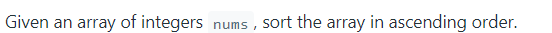• 測資的 Input/Output：
• 會拿到 nums 的變數，須回傳排序好的資料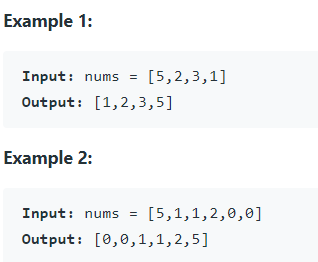• 題目的條件：

• 可能會有 1~ 50000 個數字要進行排序
• 每個數字會在 - 0.0005 ~ 50000 之間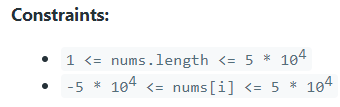• 如何利用 Quick sort 進行排序

• 上次說到 Quick Sort 有兩種實作方法，分別為

• Lomuto：兩個指標都是指向開始
• Hoare ：一個指標會指向開始，一個指標指向結尾
• 接下來我們會分別介紹兩種方式的實作

1. Lomuto

• 如昨日所提及，Lomuto 會將兩指針放於左邊。排序時，指針向右邊掃描，將小於 pivot 的元素交換於左邊，大於 pivot 的元素交換至右邊，同時可找出當前 pivot 的正確位置。
1. 首先，我們取正中間的元素作為 pivot，並設置 i, j 指針指向數列左邊：

# Partition
i, j, pivot = left, left, (left + right) // 2

其中 left 為數列左側 index， right 為數列右邊 index，以 [5, 2, 3, 1] 為例， left 為 0，而 right 為 4。下圖是初始化 i, j, 和選定 pivot。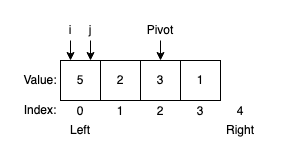1. 接著用迴圈將 i 指向比 pivot 大的元素；再將 j 指向比 pivot 小的元素

# When the loop stoped, nums[i] >= nums[pivot]
while(i < right and nums[i] < nums[pivot]): i += 1

# When the loop stoped, nums[j] < nums[pivot]
if j < i: j = i
while(j < right and nums[j] >= nums[pivot]): j += 1

由於目標是將小的元素換於左側，因此若比 pivot 小的元素本來就在左側，則不需要做任何處理，因此若 j < i 則直接讓 j = i ，繼續向右開始搜尋以節省時間。下圖是第一次掃描， i 停在 0，而 j 停在 1。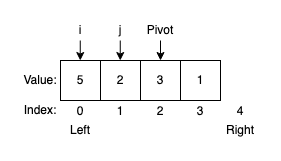2. 迴圈結束，此時 nums[i] 為比 nums[pivot] 大的元素，而 nums[j] 為比 nums[pivot] 小的元素，當 i < j (也就是 大的數 在 小的數左邊)則將兩者交換，而若有交換到 pivot ，則需更新 pivot 的位置，並將指標們 + 1 ，繼續向右掃描。下圖是交換數值，並繼續掃描。

if i < j and j < right:
# Swap
nums[i], nums[j] = nums[j], nums[i]
if i == pivot: pivot = j

# Move on
i += 1
j += 1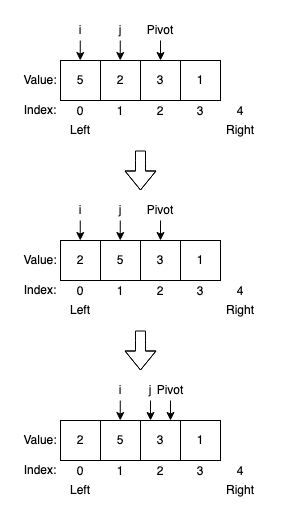3. 用迴圈重複執行 2 ~ 3 步，直到指標掃完所有元素

while(i < right and j < right)

此時數列左側都是小於 pivot 的數，而右側都是大於 pivot 的數。由於我們第二步實作大小比較時，讓 i 指向大於或「等於」pivot 的元素，因此兩側的交界就在於 i ，若先前是讓 j 指向小於或等於 pivot 的元素，則此時交界會在於 j 。下圖是掃完、交換完一輪後，指標的最終位置。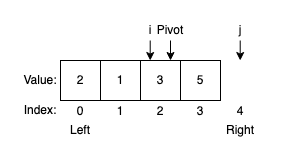4. 將 Pivot 交換至正確位置，也就是上述的交界處。下圖是將 Pivot 與 i 交換，放到正確位置。而在此例中，正好 i 和 Pivot 在同一處。

# Put pivot to the correct location
nums[i], nums[pivot] = nums[pivot], nums[i]5. 至此，我們已經成功找到一個數的正確位置，並將數列切分為小於該數的左側子數列，和大於該數的右側子數列。此處我們用遞迴方式，再將劃分出的兩個子數列繼續進行排序。下圖是經過一輪掃描，可確定 3 的正確位置在 index = 2 處，而左右可分為兩個子數列，透過遞迴繼續進行排序。

# Recursion
self.QuickSort_Lomuto(left, i)
self.QuickSort_Lomuto(i + 1, right)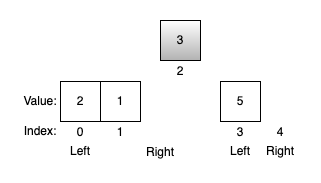6. 最後，設置遞迴函式的終止條件

# End Condition
if left >= right: return

left >= right 時停止，也就是當子數列中沒有元素時即停止。

class Solution:
def sortArray(self, nums: List[int]) -> List[int]:
self.nums = nums
self.QuickSort_Lomuto(0, len(nums))
return nums

def QuickSort_Lomuto(self, left: int, right: int) -> None:
nums = self.nums

# End Condition
if left >= right: return

# Partition
i, j, pivot = left, left, (left + right) // 2

# Sort
while(i < right and j < right):
# When the loop stoped, nums[i] >= nums[pivot]
while(i < right and nums[i] < nums[pivot]): i += 1

# When the loop stoped, nums[j] < nums[pivot]
if j < i: j = i
while(j < right and nums[j] >= nums[pivot]): j += 1

if i < j and j < right:
# Swap
nums[i], nums[j] = nums[j], nums[i]
if i == pivot: pivot = j

# Move on
i += 1
j += 1
# Put pivot to the correct location
nums[i], nums[pivot] = nums[pivot], nums[i]

# Recursion
self.QuickSort_Lomuto(left, i)
self.QuickSort_Lomuto(i + 1, right)

2. Hoare

• 首先，我們會選擇一個 pivot ，k 與 j 會逐漸靠近，k 會向右移動停在比 pivot 大的數字位置，j 會向左移動停在比 pivot 小的位置，當 k 與 j 都停止後，就做交換，直到 k 與 j 擦肩而過 ( k > j )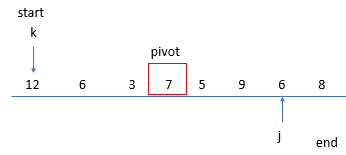• 當 k 與 j 都交換完畢，左邊就是 < pivot 的 ( start ~ j )，右邊會有 ≥ pivot 的 ( k ~ end )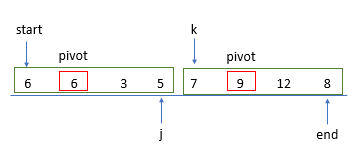• 因此，我們可以知道，每次我們會給一段要比較的開始與結尾，也就是 start 與 end，接下來就是決定 pivot，並且將比較小的放左邊，大的放右邊，不斷重複這樣的行為，需要比較的數字就會越來越小群，直到開始與結尾相同(就是剩下一個數字)，代表這個數字位置就能固定下來了
• python 實作如下
class Solution:
def sortArray(self, nums: List[int]) -> List[int]:
#       先判斷 nums 有無資料
if not nums:
return
#       開始 Quick Sort 的 Hoare 方法
self.QuickSort_Hoare(nums, 0 , len(nums) - 1)
return nums

def QuickSort_Hoare(self, nums, start, end):
#       把 start ~ end 之間要進行排序
if start >= end:
return
#       有兩個指標，k 從左邊往右，j 從右邊往左
k, j = start, end
#       pivot 為中位數
pivot = nums[(start + end) // 2]

#       當 k 與 j 會逐漸靠近，直到 k > j
while k <= j:
#           在 k 與 j 還沒相遇的情況，k 會一直往右，直到停在比 pivot 大的位置
while k <= j and nums[k] < pivot:
k += 1

#           在 k 與 j 還沒相遇的情況，j 會一直往左，直到停在比 pivot 小的位置
while k <= j and nums[j] > pivot:
j -= 1
#           在 k 與 j 還沒相遇的情況，此時 k 停在比 pivot 大的位置，j 停在比 pivot 小的位置，將兩者的值互換，並且兩個互相接近一個位置
if k <= j:
nums[k], nums[j] = nums[j], nums[k]
k += 1
j -= 1
# print(nums)
#       當 k 與 j 相遇後，位置也排完，此時 k > j，那麼 start~j 是 左邊比 pivot 小的，k ~ end 是 右邊比 pivot 大的
self.QuickSort_Hoare(nums, start, j)
self.QuickSort_Hoare(nums, k, end)